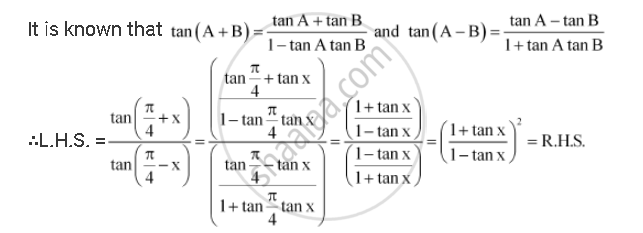CBSE (Arts) Class 11CBSE
Share

# Prove that (Tan(Pi/4 + X))/(Tan(Pi/4 - X)) = ((1+ Tna X)/(1- Tanx))Sqrt2 - CBSE (Arts) Class 11 - Mathematics

ConceptTrigonometric Functions of Sum and Difference of Two Angles

#### Question

Prove that (tan(pi/4 + x))/(tan(pi/4 - x)) = ((1+ tan x)/(1- tan x))^2

#### SolutionIs there an error in this question or solution?

#### APPEARS IN

NCERT Solution for Mathematics Textbook for Class 11 (2018 to Current)
Chapter 3: Trigonometric Functions
Q: 7 | Page no. 73

#### Video TutorialsVIEW ALL 

Solution Prove that (Tan(Pi/4 + X))/(Tan(Pi/4 - X)) = ((1+ Tna X)/(1- Tanx))Sqrt2 Concept: Trigonometric Functions of Sum and Difference of Two Angles.
S